# HOW MUCH TILE DO YOU NEED TO REMODEL A BATHROOM?##### SayHomee
###### a year ago
I believe many of you have encountered the headache of purchasing bathroom tiles in home renovation. The responsible contractors will calculate how much tile you need, while some may leave you helpless in this situation. So, how to accurately calculate the tile quantity and the coverage area to meet your demand without wasting material? Keep reading.

Subribe our newsletter to receive the latest articles and home renovation ideas directly in your email inbox. We will only send interesting ideas we like!
Or
By subscribing, you agree to our privacy and agreement terms.

I believe many of you have encountered the headache of purchasing bathroom tiles in home renovation. The responsible contractors will calculate how much tile you need, while some may leave you helpless in this situation. So, how to accurately calculate the tile quantity and the coverage area to meet your demand without wasting material? Keep reading.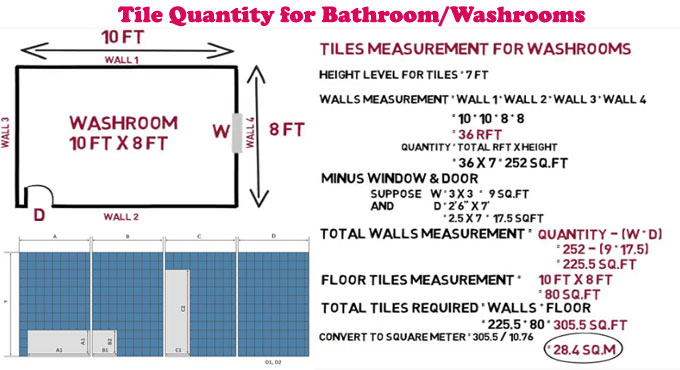#### Preparation

• Paper
• Writing tools
• A calculator
• A tape measurer
• Photos of the room (optional)

#### Steps:

This task is very simple. In this blog, we demonstrate three examples on how to calculate the tile quantity for the floor, bathroom backsplash, and showers with mirrors.

• Paint or print the photos of said space
• Measure the room and mark the graphs or photos using inch as unit
• If necessary, please section the entire space, and mark them with letter A to Z to avoid confusions
• Multiply the height and width of each section
• Add all sections together and divide the number by 144 to get the square footage
• Subtract the windows and benches from the total number
• Add an extra area of 10%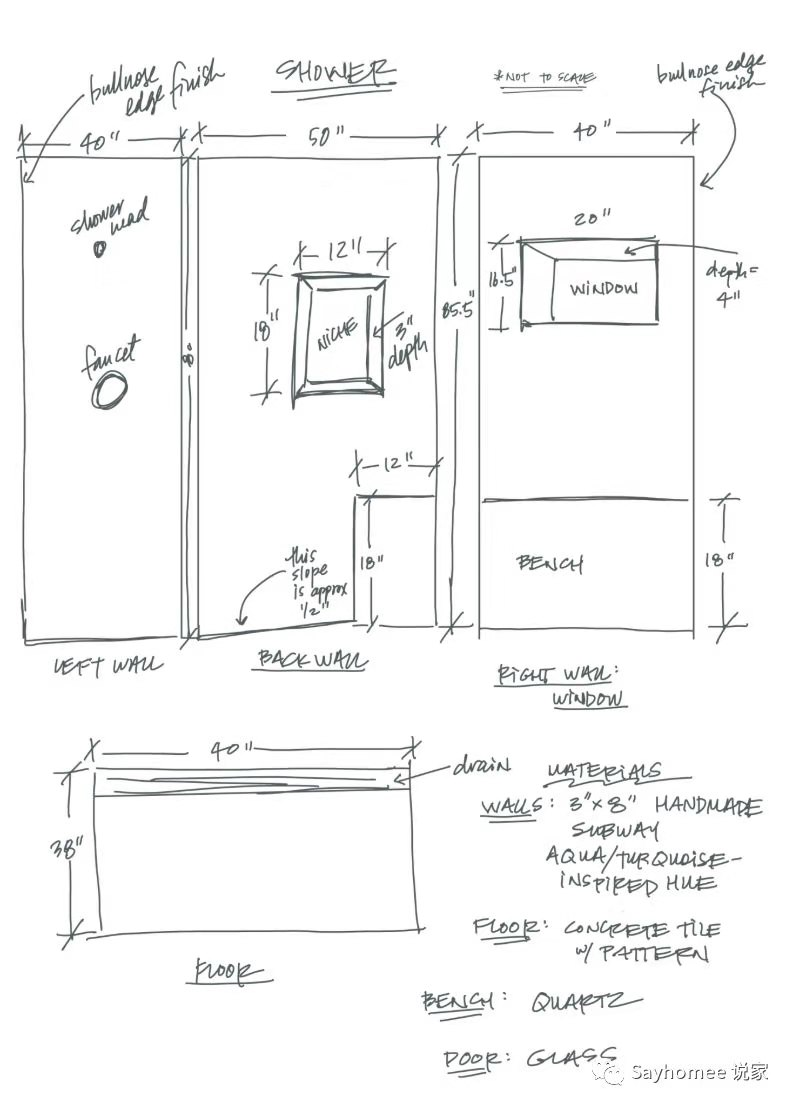##### Example 1: Floor

The floor is the easiest part, all we need is a section A, and the following is the formula:

• Total Area: 36” x 36” = 1,296
• Convert to Square Feet: 1,296/144 = 9 sf
• (Add some extra) 9 x 1.2 = 10.8 sf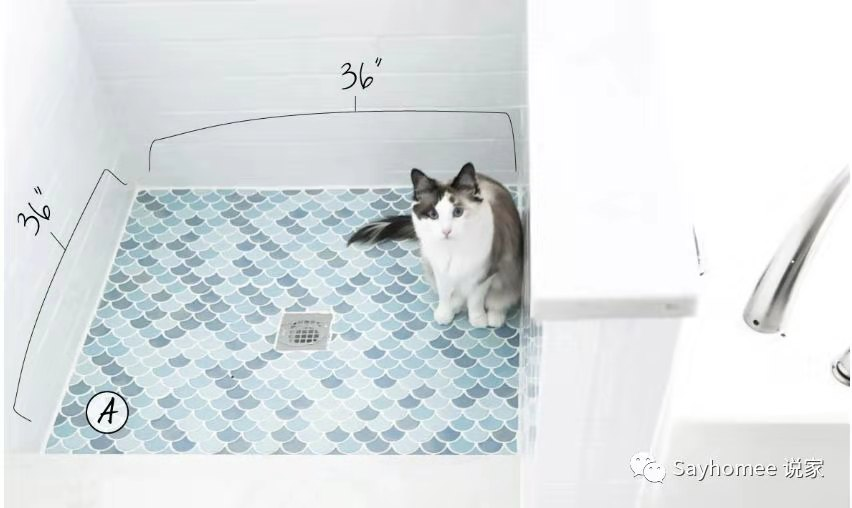Factors may affect the result: drains, sinks, toilets, or other subjects that take a lot of space. Most items will be removed in demolish. And we would always recommend installing tiles under items for future renovation. If there are things that cannot be removed, you can section the floor into two.

##### Example 2: Bathroom Backsplash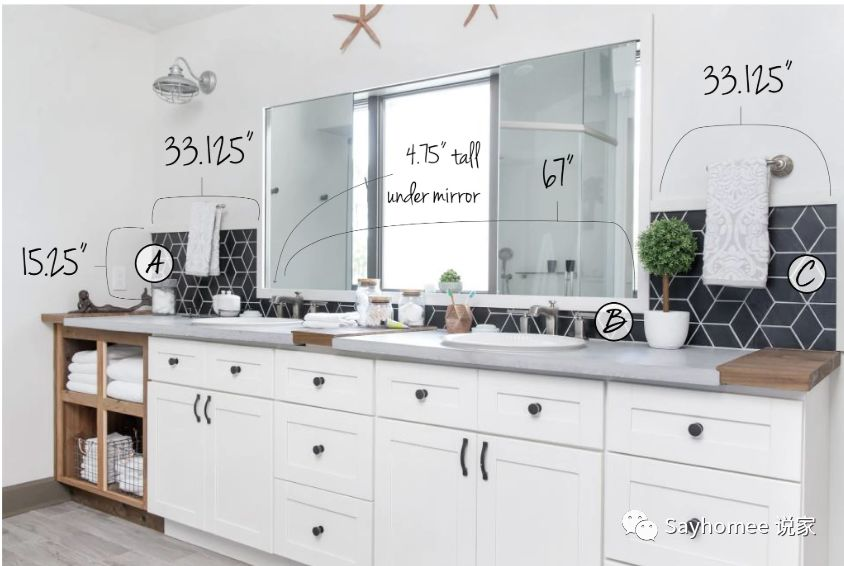There is usually a mirror on the bathroom backsplash to separate the rectangular into multiple sections. And this is how we paint and measure the square footage.

• Section A = 15.25“ x33.125” = 505.16
• Section B = 4.75“ x67” = 318.25
• Section C = 15.25“ x33.125” = 505.16

Total Area

• Section A + Section B + Section C = 1,328.57
• 1,328.57/144 = 9.2 sf
• (Add the extra) 9.2x1.1 = 10.12 sf
##### Example 3: How to Calculate the Shower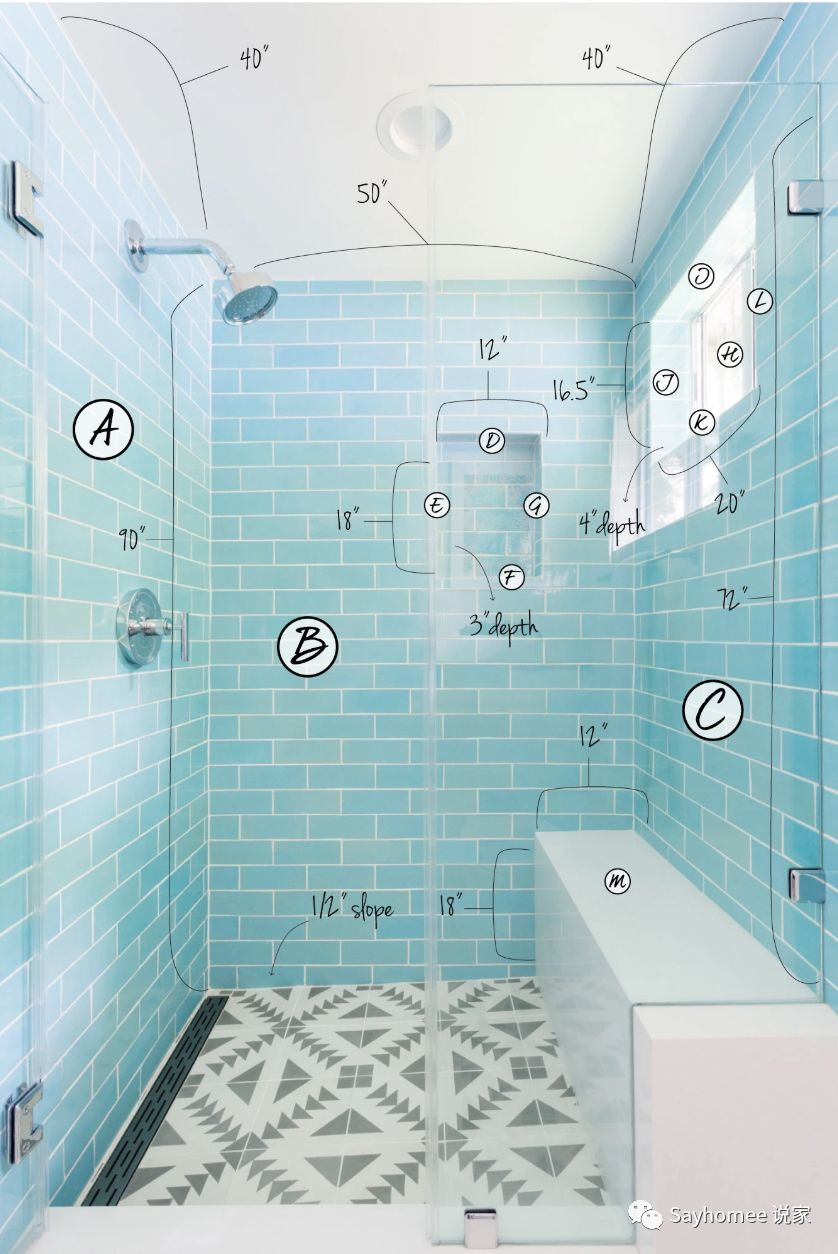• Section A = 90“x40” = 3,600“
• Section B = 90“x50” = 4,500“
• Section C = 72“x40” = 2,880“
• Section D = 12“x3” = 36“
• Section E = 18“x3” = 54“
• Section F = 12“x3” = 36“
• Section G = 18“x3” = 54”
• Section H = 16.5“x20” = 330“
• Section I = 20“x4” = 80“
• Section J = 16.5“x4” = 66“
• Section K = 20“x4” = 80“
• Section L = 16.5“x4” = 66“
• Section M = 18“x12” = 216“

Total Area

• Add all sections besides the station and the window (Section M and H) = 11,452
• Subtract the sections without tiles (Section M and H): 11,452 – 330 – 216 = 10,906
• 10,906/144 = 75.7 sqft
• 75.7x1.1 = 83.3 sqft

#### Summary

Above is the commonly used method to calculate the tile quantity. If you do not want to spend too much time on it, you may calculate with just the height, length, and width, without counting some small areas.

The kitchen and other rooms are calculated the same way too. You should feel confident to calculate the tile quantity for any rooms by now.

If you still have concerns, please contact our customer representatives below, and we will provide you with more details.